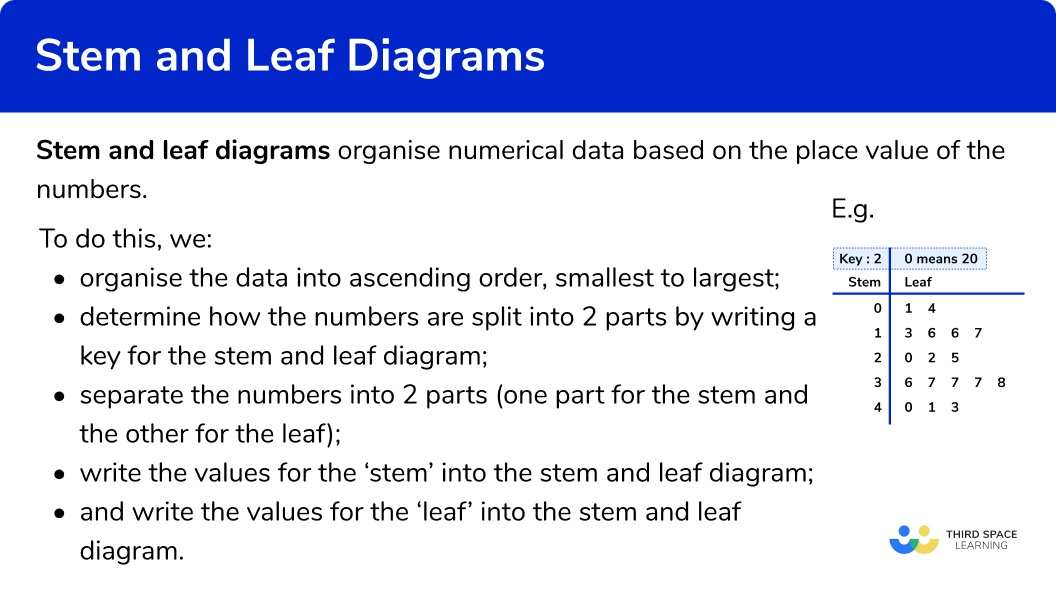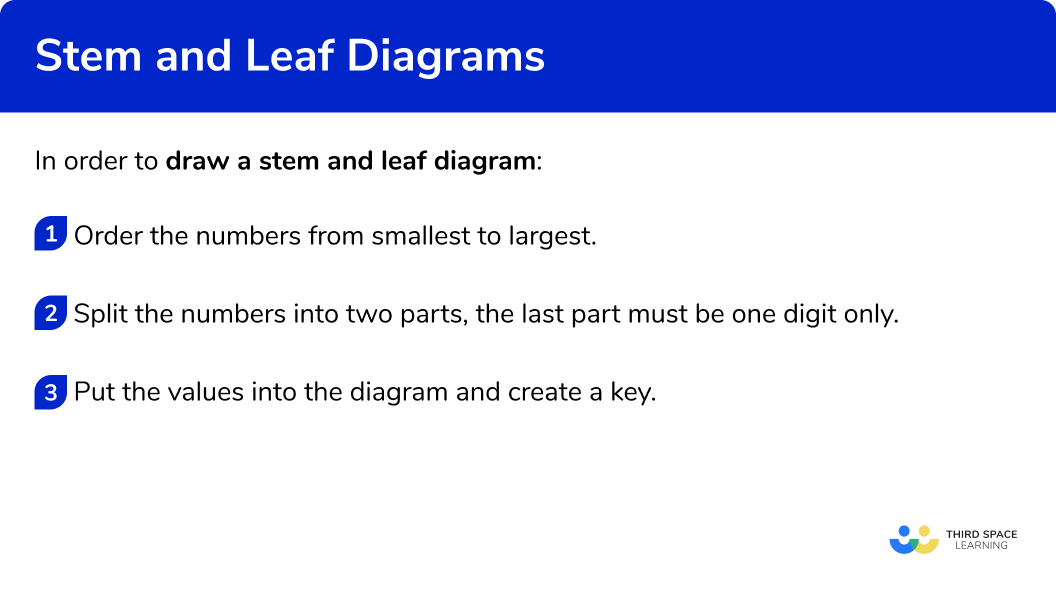GCSE Maths Statistics Representing Data

Stem And Leaf Diagram

# Stem And Leaf Diagram

Here we will learn about stem and leaf diagrams, including drawing, interpreting and comparing diagrams.

There are also stem and leaf diagram worksheets based on Edexcel, AQA and OCR exam questions, along with further guidance on where to go next if you’re still stuck.

## What is a stem and leaf diagram?

A stem and leaf diagram is a method of organising numerical data based on the place value of the numbers.

Each number is split into two parts.

• The first digit(s) form the stem,
• The last digit forms the leaf.

The leaf should only ever contain a single digit.

For example, the number 154 would be split into two parts where the digits \bf{15} would be the stem and \bf{4} would be the leaf.

To set up a stem and leaf diagram we need to:

• Organise the data into ascending order, smallest to largest;
• Determine how the numbers are split into 2 parts by writing a key for the stem and leaf diagram;
• Write the values for the ‘stem’ into the stem and leaf diagram;
• Write the values for the ‘leaf’ into the stem and leaf diagram.

Note: A stem and leaf diagram must have a key (sometimes referred to as a legend).  This explains how to convert the digits in the stem and leaf diagram into a single data point. Remember to include any units in the key if appropriate.

We can use stem and leaf diagrams to calculate averages like the median, the mode and the mean, and to calculate measures of spread like the range and the interquartile range.

### What is a stem and leaf diagram?### Dual stem and leaf diagram

Comparing data sets is simplified by using a dual stem and leaf diagram which have two sets of data represented back to back.

For example, here are two sets of data showing test scores of 20 males and 20 females.

By combining them together to form one dual stem and leaf diagram, we can directly compare the two sets of data.

The data must be closely related for it to be compared effectively on the diagram.

Note that the digits in the leaf for Females is still in ascending order but from right to left, rather than left to right. Also, the key represents the data values for each side of the stem and leaf diagram.

## How to draw a stem and leaf diagram

In order to draw a stem and leaf diagram:

1. Order the numbers from smallest to largest.
2. Split the numbers into two parts, the last part must be one digit only.
3. Put the values into the diagram and create a key.

### How to draw a stem and leaf diagram### Related lessons on representing data

Stem and leaf diagram is part of our series of lessons to support revision on representing data. You may find it helpful to start with the main representing data lesson for a summary of what to expect, or use the step by step guides below for further detail on individual topics. Other lessons in this series include:

## Stem and leaf diagram examples

### Example 1: two digit numbers

The data below shows the ages of people at a party.

Draw an ordered stem and leaf diagram for the data. Remember to include a suitable key.

1. Order the numbers from smallest to largest.

becomes

2Split the numbers into two parts, the last part must be one digit only.

The number in our data will split into tens and units so 35 will be 3 and 5 \; (3 represents 30 and 5 is 5 units)

3Put the values into the diagram and create a key.

### Example 2: three digit numbers

The data below shows the length in centimetres of 13 long jumps on a year 9 sports day.

Draw an ordered stem and leaf diagram for the following set of data.  Remember to include a suitable key.

Order the numbers from smallest to largest.

Split the numbers into two parts, the last part must be one digit only.

Put the values into the diagram and create a key.

### Example 3: decimal values

A group of students are making model gargoyles out of clay. The weight of each gargoyle is written below.

Draw an ordered stem and leaf diagram for data.  Remember to include a suitable key.

Order the numbers from smallest to largest.

Split the numbers into two parts, the last part must be one digit only.

Put the values into the diagram and create a key.

### Example 4: answering questions using a stem and leaf diagram

The stem and leaf diagram below shows the ages of a group of people at a party.

How many people are there in the group?

What age is the youngest member of the group?

What age is the oldest member of the group?

How many people are under \bf{45} ?

How many people are \bf{45} and over?

### Example 5: calculating averages and the range from a stem and leaf diagram

The stem and leaf diagram below shows the weight of 10 puppies in kilograms

Write down the modal weight.

Find the median weight.

Find the range of the weights.

Find the mean weight of the puppies.

### Example 6: dual stem and leaf diagram

This dual stem and leaf diagram shows the results for the students in Miss Thomas’ class.

Find the range of the boys’ results.

Find the median of the girls’ results.

Find the modal mark for the boys’ results.

Compare the boys’ and girls’ results.

### Common misconceptions

• Incorrectly reading the numbers in the diagram

For example, for the following stem and leaf diagram, the value of 13\rvert5 is misinterpreted as the value 5, instead of 135 .

• Miscounting

A common error is to incorrectly count the numbers in a stem and leaf diagram when calculating an average or range. It is a good idea to mark off each number to avoid missing a number out

• More than one digit for the leaf

The leaf must only be one digit, otherwise the data can be greatly misinterpreted. Make sure that the smallest place value for the data is used for the leaf.

### Practice stem and leaf diagram questions

1. Which stem and leaf diagram matches this data?

Use the key   2 \rvert 1 \text{ represents } 21 cm .By checking the highest and lowest values from the list ( 29 is the lowest and 63 is the highest) we can see that the correct stem and leaf is2. The stem and leaf diagram below shows heights of Mrs Wilks’ flowers in centimetres.What is the range of the height of Mrs Wilks’ flowers?

8223241The range is found by subtracting the lowest value from the highest value. 41-9 = 32cm

3. Below is a stem and leaf diagram showing the scores of 18 javelin throws in a school sports day. The longest throw wins.What was the mean distance thrown?

10m19.9m20.5m3.8mThe mean is the sum of all the values, divided by the total number of values.

5+8+9+10+10+14+16+17+20+21+22+25+26+29+30+31+32+34=359m

359 \div 18=19.9\overline{4}m=19.9m \; (1dp)

4. The stem and leaf diagram below represents a sample of the lengths of leaves from a tree.Which average would be the most suitable to represent the data?

MeanMedianRangeModeAs there is one value that is very large, this would affect the mean and the range. The mode is not suitable because it does not use all of the data.

The median is most suitable as this is not affected as much as the mean when we have more extreme values.

5. The stem and leaf diagram below shows the maximum weight of a deadlift lifted by each competitor in a weightlifting competition.

Everyone managed to record at least one lift.What was the median weight lifted?

215lbs220lbs225lbs235lbsThe median is located at the middle position in the data. 17 \div 2=8.5^{th} value. This value lies between 220lbs and 220lbs.As these two values are the same, the median is 220lbs .

6. 42 people competed in a local 3 legged race. The time for each pair was recorded in a back to back stem and leaf diagram.Select the completed stem and leaf diagram for the adult race using the results below.The values are written:

• In the correct row
• In order (right to left) from the stem
• On the left hand side of the stem and leaf diagram### Stem and leaf diagram GCSE questions

1. Here are the ages of 14 people in years.

Draw an ordered stem and leaf diagram for their ages.

(3 marks)For all values in the stem and the leaf that match the data

(1)

For numbers in ascending order

(1)For a suitable key

(1)

2. The number of people visiting a restaurant each day, for 11 days, is listed below.

(a) Use the key to complete an ordered stem and leaf diagram for this information.(b) Write down the mode.

(c) Write down the median

(d) Write down the range.

(5 marks)

(a)Data ordered smallest to largest

(1)

Numbers correctly inputted into the stem and leaf diagram

(1)

(b) 120

(1)

(c) 120

(1)

(d) 131-104 = 27

(1)

3. The head of modern languages is looking at the year 11 mock exam results for German and Spanish.

The results are shown below in the stem and leaf diagram:(a) What is the modal score for Spanish?

(b) What is the modal group for German?

(c) What is the difference between the range for German and the range for Spanish?

(d) Compare the median value for the two subjects and comment on the pupils’ performance.

(6 marks)

(a) 57

(1)

(b) 70-79

(1)

(c)

Median (German) = 65, Median (Spanish) = 60

(1)

Range (German) =45 , Range (Spanish) =57

(1)

57-45=12

(1)

(d) The median for German is greater than the median for Spanish suggesting that the pupils achieved better overall in German.

(1)

## Learning checklist

You have now learned how to:

• Interpret and construct tables
• Construct and interpret diagrams for discrete data
• Interpret, analyse and compare the distributions of data sets from univariate empirical distributions through appropriate graphical representation involving discrete data

## Still stuck?

Prepare your KS4 students for maths GCSEs success with Third Space Learning. Weekly online one to one GCSE maths revision lessons delivered by expert maths tutors.

Find out more about our GCSE maths tuition programme.